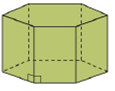Chapter 9.2, Problem 2EElementary Geometry For College St...

7th Edition
Alexander + 2 others
ISBN: 9781337614085

Solutions

Chapter
SectionElementary Geometry For College St...

7th Edition
Alexander + 2 others
ISBN: 9781337614085
Textbook Problem

In Exercises 1 to 4, name the solid that is shown. Answers are based on Sections 9.1 and 9.2.a)Bases are regular.b)Bases are not regular.

To determine

(a)

To name:

The solid in the given figure.

Explanation

Given:

The figure given below

and bases are regular.

Properties Used:

A prism is a polyhedron, which means all faces are flat and the side faces are parallelograms and each one is called a base. It has the same cross section all along its length.

A right prism is a prism in which the lateral edges are perpendicular to the base edges at their point of intersection.

An oblique prism is a prism in which the parallel lateral edges are oblique to the base edges at their point of intersection...

To determine

(b)

To name:

The solid in the given figure.

Still sussing out bartleby?

Check out a sample textbook solution.

See a sample solution

The Solution to Your Study Problems

Bartleby provides explanations to thousands of textbook problems written by our experts, many with advanced degrees!

Get Started

Find more solutions based on key concepts